Torque Sensor 1: Mechanicals of Materials

Torque Sensor 2: Strain Gauge & Wheatstone Bridge

Torque Sensor 3: Instrumentation Amplifier

Torque Sensor 4: Torque Sensor Build Log

Updated: 5/15/2016 – Initial Page Creation

Okay, I debated making this page because I really want to make torque sensors and sell them, and this page will pretty much detail all of the engineering that went into designing my torque sensor. But in the end, I decided it was more important to share the information I have gathered than any possible product sales I may lose from people making their own instead of buying mine. And that is the premise behind this website, sharing information I have gathered in the same way hundreds of thousands of others have done before me. Anyways, let’s get started on the torque sensor.

Quick Mechanics of Materials Review:
In order to get into the equations governing our torque sensor design, we need to cover the math calculating torsion. First of all, torsion is when a shaft is twisted because of a torque applied to it. When a shaft is under a torque load, the torsion throughout the cross section of the shaft increases linearly from the center of the shaft to the outer surface – see the picture below for details.The equation for the shear stress in the shaft is given as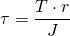where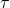is the shear stress, T is the torque, r is the radius, and J is the polar moment of inertia. So we need to know how to calculate sheer strain from the sheer stress, and how to calculate the polar moment of inertia. The polar moment of inertia can be looked up online, the two most common shapes are the solid shaft and the hollow shaft. The polar moment of inertia for these shapes is shown below:

For a solid shaft:For a hollow shaft: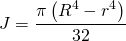Now from the basic definition of stress and strain, we know that stress is equal to the strain multiplied by the elastic modulus. In the case of torsion, the value used is called the “Shear Modulus” – this value can be found online, but it may be easier to calculate it from the Elastic Modulus and Poisson’s Ratio of the material.
In equation form:

Shear Strain: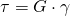where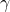is the shear strain, and G is the Shear Modulus

Shear Modulus: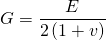where E is the Elastic Modulus (or Young’s Modulus or Modulus of Elasticity)

Okay, so let’s solve all of these equations for the shear strain of a given shaft of a given material with a given torque.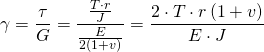For a solid shaft:For a hollow shaft:Let’s try an example:
I want to measure torque applied to a socket extension bar. I know that the torque range I will apply is from 0 to 140 N-m. The shaft is approximately 12mm in diameter and is a solid shaft. The material is Chrome Molybdenum Alloy steel – I don’t know for sure but let’s just say it is 4130 steel. Looking up the properties yields 205 GPa for the modulus of elasticity – GPa is GigaPascal, or one billion Pascals, Pascal is one Newton per square meter. Poisson’s Ratio for this material is 0.29. Let’s plug these into the equation!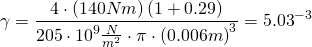You may notice that the strain has no units – that is true since the definition of strain is a change in value – it is technically a percent. I’ll cover that more later.

Torque Sensor 1: Mechanics of Materials
Tagged on: